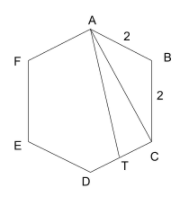# CAT 2021 Question Paper | Quant Slot 1

###### CAT Previous Year Paper | CAT Quant Questions | Question 15

CAT 2021 Quant was dominated by Arithmetic followed by Algebra. In Arithmetic, the questions were dominated by topics like Speed-time-distance, Mixture and Alligations. This year, there was a surprise. The questions from Geometry were relatively on the lower side as compared to the previous years. There were 8 TITA Qs this year. Overall this section was at a medium level of difficulty.

Question 15 : Suppose the length of each side of a regular hexagon ABCDEF is 2 cm. It T is the mid point of CD, then the length of AT, in cm, is

1. √15
2. √13
3. √12
4. √14

## Best CAT Coaching in Chennai

#### CAT Coaching in Chennai - CAT 2022Limited Seats Available - Register Now!In a regular hexagon, each internal angle is equal to 120°.
From isosceles triangle ABC, we know the length of two sides and including angle.
We will be able to find the third side (AC) using the Pythagoras theorem or the sine rule.
Hence, AC = 2√3cm
Given that, T is the midpoint.
So, CT = 1cm.
From the right-angled △ ACT,
AC2 + CT2 = AT2
AT2 = (2 √3)2 + (1)2 = 13
AT = √(13)

The question is " Suppose the length of each side of a regular hexagon ABCDEF is 2 cm. It T is the mid point of CD, then the length of AT, in cm, is "

##### Hence, the answer is '√13'

Choice B is the correct answer.

###### CAT Coaching in ChennaiCAT 2022

Classroom Batches Starting Now! @Gopalapuram

###### Best CAT Coaching in Chennai Introductory offer of 5000/-

Attend a Demo Class

##### Where is 2IIM located?

2IIM Online CAT Coaching
A Fermat Education Initiative,
58/16, Indira Gandhi Street,
Kaveri Rangan Nagar, Saligramam, Chennai 600 093

##### How to reach 2IIM?

Phone: (91) 44 4505 8484
Mobile: (91) 99626 48484 / 94459 38484
WhatsApp: WhatsApp Now
Email: info@2iim.com